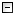Home Control Panel Login Register Search Submit Logout About me Contact meMy ResearchWeb This Site
 Home > SAS WinBUGS for factor analysis
SAS WinBUGS for factor analysis
 SAS WinBUGS for factor analysis /*Because of reflection effect of factor analysis (identification problem), one may find that the estimated factor loadings could have opposite signs. (It only occurs occasionally and thus could be hard to notice.) To avoid this problem, one can constrain the factor loading > 0. This can be done easily in WinBUGS. Note that the codes on this page, we added I(0,) in the priors of the factor loadings. However, users should be careful to put this kind of constraints. Another way to avoid this problem is to fix one of the factor loadings to be a known constant and estimate the factor variance. */ TITLE 'Run WinBUGS from SAS: A confirmatory factor Example by Zhang et al. (2006)'; /*WinBUGS program for CFA*/FILENAME model "C:\zzy\research\SASWinBUGS\cfamodel.txt";DATA model;INPUT model \$80.;CARDS;/*start the model*/model{for (i in 1:N) { for (t in 1:T){ y[i,t]~dnorm(muy[i,t],Inv_sig2[t]) muy[i,t]<-fload[t]*fscore[i]}fscore[i]~dnorm(0, 1)} for (t in 1:T){ fload[t]~dnorm(0, 1.0E-6)I(0,)Para[t]<-fload[t] Inv_sig2[t]~dgamma(0.001, .001)Para[t+4]<-1/Inv_sig2[t]} } ;RUN;DATA _NULL_; SET model; FILE model; PUT model;RUN; /*Starting values*/DATA _NULL_;FILE "C:\zzy\research\SASWinBUGS\cfaini.txt";PUT "list(fload=c(.5,.5,.5,.5), Inv_sig2=c(.5,.5,.5,.5))";RUN; /*Scripts to run WinBUGS*/FILENAME runcfa 'c:\program files\winbugs14\runcfa.txt';DATA _NULL_; FILE runcfa; PUT@1 "display('log')"; PUT@1 "check('C:/zzy/research/SASWinBUGS/cfamodel.txt')" ; PUT@1 "data('C:/zzy/research/SASWinBUGS/cfadata.txt')"; PUT@1 "compile(1)"; PUT@1 "inits(1, 'C:/zzy/research/SASWinBUGS/cfaini.txt')"; PUT@1 "gen.inits()"; PUT@1 "update(2000)"; PUT@1 "set(Para)"; PUT@1 "update(3000)"; PUT@1 "stats(*)"; PUT@1 "save('C:/zzy/research/SASWinBUGS/cfalog.txt')"; PUT@1 "quit()";RUN; DATA _NULL_;FILE "C:\zzy\research\SASWinBUGS\runcfa.bat";PUT '"C:\program files\WinBUGS14\WinBUGS14.exe" /PAR runcfa.txt';PUT 'exit';RUN; %macro simcfa(n);TITLE2 'Generate the Data';DATA Sim_CFA;*setting the true parameter values;fload=.8; sig2=.36;* setting statistical parameters; N = 200; seed = 20060802+&n; M=4;* need to setup arrays so we can have more variables;ARRAY y_score{4} y1-y4;ARRAY e_score{4} y1-y4; * generating raw data; DO _N_ = 1 TO N;* now the indicator variables ; f_score=RANNOR(seed); DO t = 1 TO M; y_score{t} = fload*f_score +sqrt(sig2)*RANNOR(seed); END; KEEP y1-y4; OUTPUT; END;RUN; /*Data*/%_sexport(data=Sim_CFA, file='C:\zzy\research\SASWinBUGS\cfadata.txt',var=y1-y4); /*Run WinBUGS*/DATA _NULL_;X "C:\zzy\research\SASWinBUGS\runcfa.bat";RUN;QUIT; /*Read in the log file to view the DIC*/TITLE2 'Simulation '&n;DATA log;INFILE "C:\zzy\research\SASWinBUGS\cfalog.txt" TRUNCOVER ;INPUT log \$80.;log=translate(log," ","09"x);IF (SUBSTR(log, 2, 4) ne 'Para') then delete;RUN; PROC PRINT DATA=log;RUN;%mend; %macro runsimcfa;   %let n=1;      %do %while(&n <= 100);         %simcfa(&n);      %let n=%eval(&n+1);   %end;%mend runsimcfa; %runsimcfa; DM OUTPUT 'FILE "C:\zzy\research\SASWinBUGS\allresults.txt"';DM LOG    'FILE "C:\zzy\research\SASWinBUGS\allresults.log"'; /*Analyze the results*/DATA temp;INFILE "C:\zzy\research\SASWinBUGS\allresults.txt" TRUNCOVER ;INPUT all \$90.;IF (SUBSTR(all, 7, 4) NE 'Para') THEN DELETE;FILE "C:\zzy\research\SASWinBUGS\temp.txt";PUT all;RUN; /*To avoid the reflection effect, one can also convert the negative loadings to positive here*/ DATA temp;INFILE "C:\zzy\research\SASWinBUGS\temp.txt";INPUT parid parname \$ parest parsd MCerror p25 median p975 start sample;id=int((_N_-.1)/8)+1;parest=abs(parest);RUN; /*Parameter Estimates*/PROC TRANSPOSE DATA=temp OUT=parest PREFIX=par;    BY id ;    ID parid;    VAR parest;RUN; PROC MEANS DATA=parest;VAR par1-par8;RUN; /*SDs*/PROC TRANSPOSE DATA=temp OUT=parsd PREFIX=sd;    BY id ;    ID parid;    VAR parsd;RUN; PROC MEANS DATA=parsd;VAR sd1-sd8;RUN; Submitted by: johnny1 2 3 4 5 6 7 8 9 10
 Copyright © 2003-13 Zhiyong Zhang \'s Psychometric WebsiteMaintained by Zhiyong Zhang